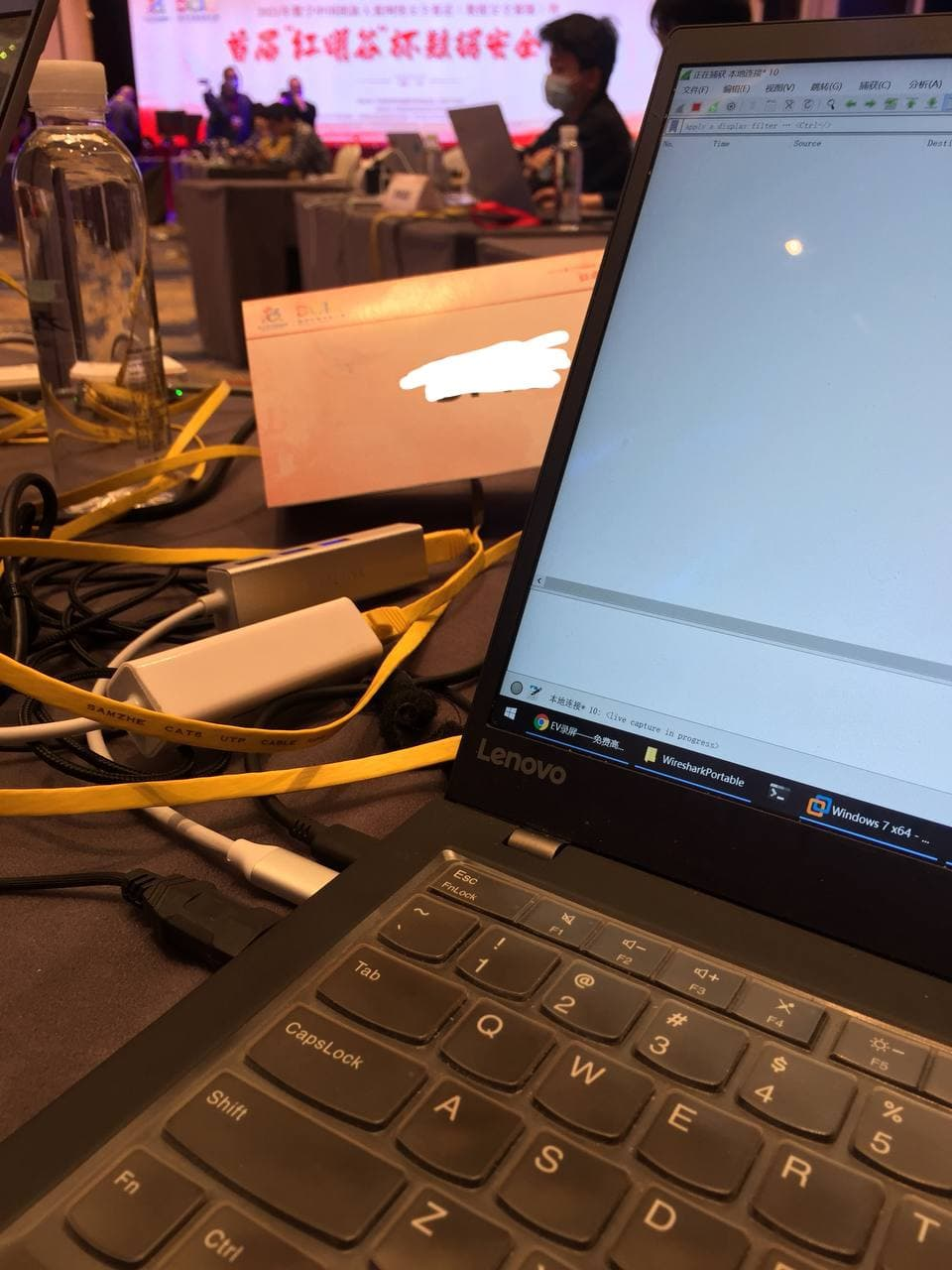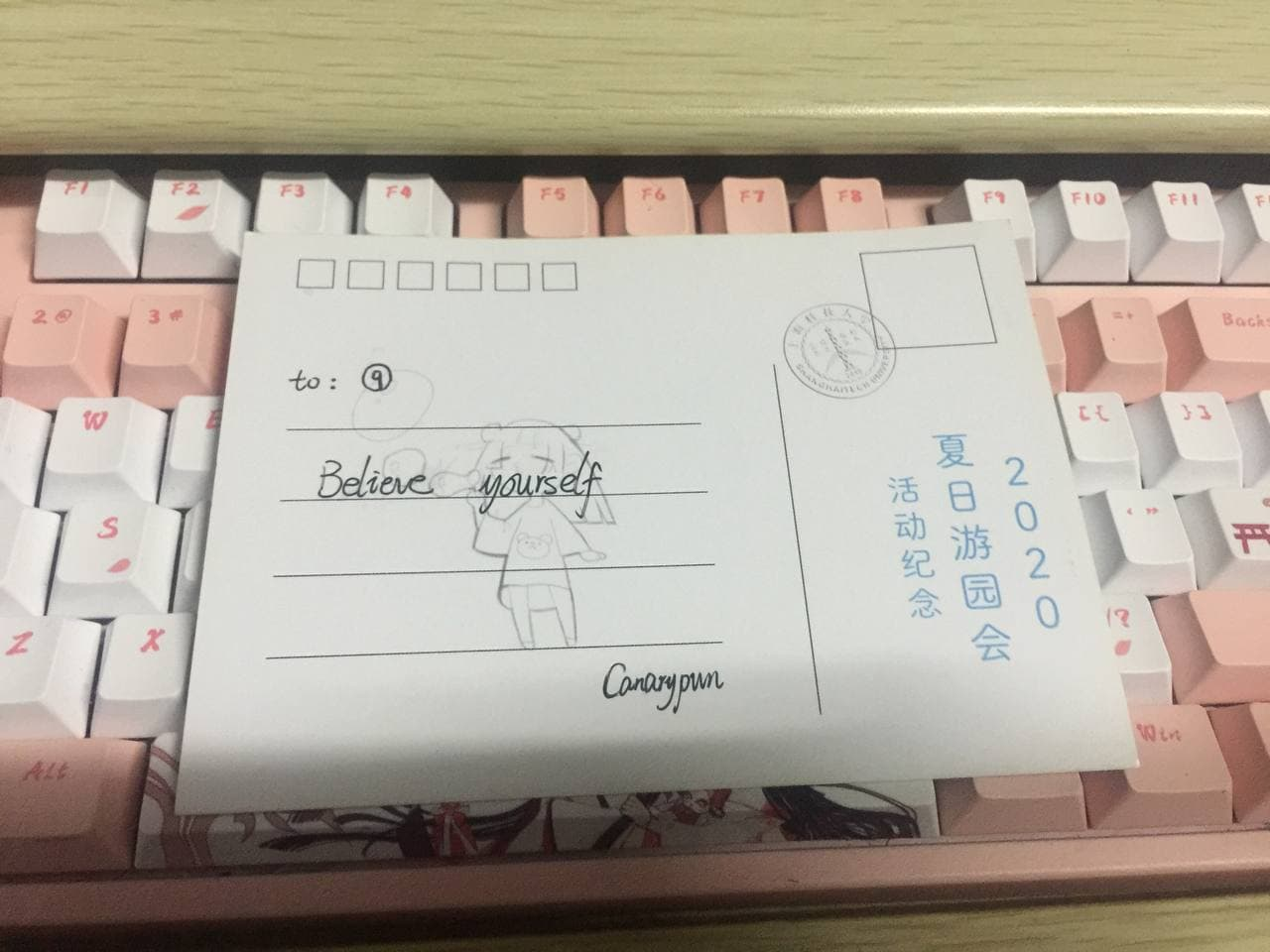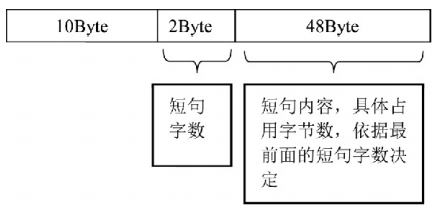﻿ ⑨BIE » ctf

# 前言# 经过（不是俺的手）

# 题外话Tags: ctf, 线下

# 前言

`C:\Users\username\AppData\Roaming\Microsoft\InputMethod\Chs` 下有两个`ChsPinyinUDL.dat``ChsPinyinIH.dat`文件里面有记录词频信息。``````f = open("ChsPinyinUDL.dat","rb")
data = data[9216:]
f.close()
i = 60
n=1
while True:
chunk = n*i
chunk_len = data[chunk+12:chunk+12+48]
hex_chunk_len = ['%02x' % b for b in chunk_len]
print(chunk_len.decode("utf-16"))
n+=1
if chunk>=len(data):
break
``````
``````f = open("ChsPinyinIH.dat","rb")
data = data[5120:]
f.close()
i = 60
n=1
while True:
chunk = n*i
chunk_len = data[chunk:chunk+4]
hex_chunk_len = ['%02x' % b for b in chunk_len]
print(hex_chunk_len[::-1])
unicode_chunk = data[chunk+12:chunk+12+data[chunk]*2]
hex_unicode = ['%02x' % b for b in unicode_chunk]
print(hex_unicode[::-1])
print(unicode_chunk.decode("utf-16"))
n+=1
if chunk>=len(data):
break``````Tags: ctf, 取证

## 时隔许久的ctf感想

AWD倒是真的没怎么打过Hhhh这次过去貌似真的只能划水了23333

Tags: ctf